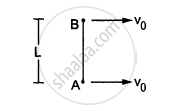Department of Pre-University Education, KarnatakaPUC Karnataka Science Class 11
Advertisement Remove all ads

# Two Small Balls a and B, Each of Mass M, Are Joined Rigidly to the Ends of a Light Rod of Length L (See the Following Figure). - Physics

Sum

Two small balls A and B, each of mass m, are joined rigidly to the ends of a light rod of length L (see the following figure). The system translates on a frictionless horizontal surface with a velocity $\nu_0$ in a direction perpendicular to the rod. A particle P of mass m kept at rest on the surface sticks to the ball A as the ball collides with it. Find
(a) the linear speeds of the balls A and B after the collision, (b) the velocity of the centre of mass C of the system A + B + P and (c) the angular speed of the system about C after the collision.[Hint : The light rod will exert a force on the ball B
only along its length.]

Advertisement Remove all ads

#### Solution

(a) Collision will not affect the velocity of ball B because the light rod will exert a force on it only along its length.

Therefore, we have

Velocity of B = v0

For ball A,

On applying the law of conservation of linear momentum, we get

$m \nu_0 = 2m \times \nu$

$\Rightarrow v = \frac{v_0}{2}$

$\therefore$ Velocity of A $= \frac{\nu_0}{2}$

(b) If we consider the three bodies to be a system, we have

Net external force = 0

$v_{CM} = \frac{m \times v_0 + 2m \times \left( v_0 /2 \right)}{n + 2m}$

$= \frac{m v_0 + m v_0}{3m}$

$= \frac{2 v_0}{3}$

(Direction will be same as the initial velocity before collision.)

(c) Velocity of (A + P) w.r.t. the centre of mass $= \left\{ \frac{2 v_0}{3} - \frac{v_0}{2} \right\} = \frac{v_0}{6}$

Velocity of B w.r.t. the centre of mass $= v_0 - \frac{2 v_0}{3} = \frac{v_0}{3}$

Distance of the (A + P) from the centre of mass $= \frac{l}{3}$

Distance of the B from the centre of mass = $\frac{2l}{3}$

Applying  $m v_{com} r = l_{cm} \times \omega,$ we  have

$\left( 2m \times \frac{v_o}{6} \times \frac{l}{3} \right) + \left( m \times \frac{v_0}{3} \times \frac{2l}{3} \right) = \left\{ 2m \left( \frac{l}{3} \right)^2 + \left( \frac{2l}{3} \right)^2 m \right\} \times \omega$

$\Rightarrow \frac{6m v_0 l}{18} = \frac{6ml}{9}\omega$

$\Rightarrow \omega = \frac{v_0}{2l}$

Is there an error in this question or solution?
Advertisement Remove all ads

#### APPEARS IN

HC Verma Class 11, 12 Concepts of Physics 1
Chapter 10 Rotational Mechanics
Q 63 | Page 199
Advertisement Remove all ads
Advertisement Remove all ads
Share
Notifications

View all notifications

Forgot password?